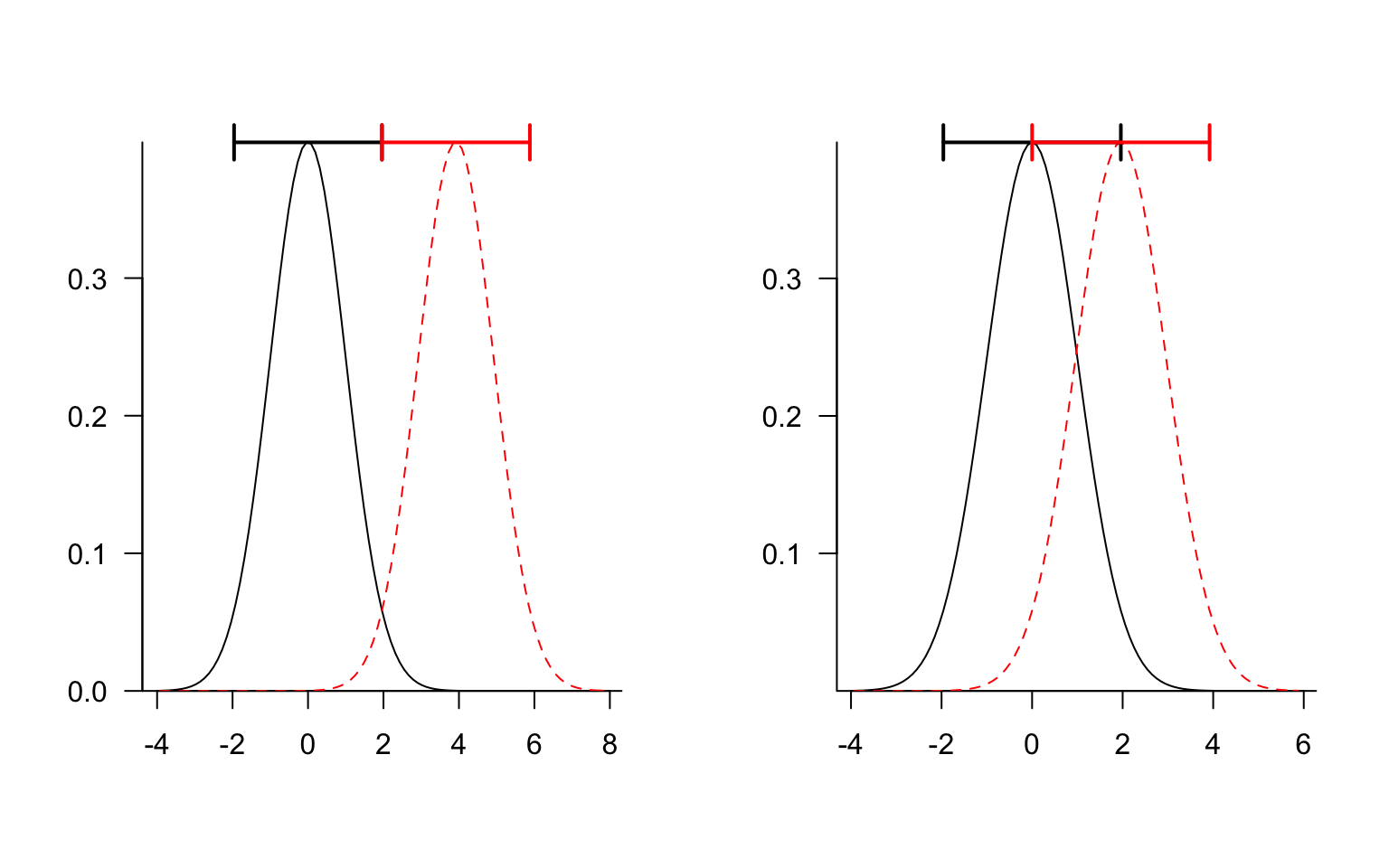Case I: Consider two Normal distributions, both with $$\sigma=1$$, with means 0 and $$2 \times 1.96 = 3.92$$, so that their 95% CIs ($$\pm 1.96$$) just touch.

Let’s set $$\phi=1.96$$ for notational convenience, and assume that the two values are independent. Then the variance of their difference $$(\mu_1-\mu_2)$$ is $$\sigma^2_1 + \sigma^2_2 = 2$$; the standard deviation of the difference is $$\sqrt{2}$$; the $$Z$$-statistic is $$2 \phi/\sqrt{2} = 2.77$$; and the $$p$$-value (0.0056) is considerably less than 0.05, so this is a conservative criterion.

A brief Google scholar search shows that this has been rediscovered/discussed many times (Goldstein and Healy 1995; Payton, Miller, and Raun 2000; Schenker and Gentleman 2001; Austin and Hux 2002; Wolfe and Hanley 2002; Payton, Greenstone, and Schenker 2003; Cumming and Finch 2005; Knezevic 2008; Cumming 2009; MacGregor-Fors and Payton 2013)!

Case II: Now let’s suppose that $$\mu_2$$ is only $$\phi$$ rather than $$2 \phi$$, i.e. that the 95% upper confidence limit of the first group just touches the estimate for the second group (rather than the 95% lower confidence limit for the second group). Now the $$Z$$-statistic is $$\phi/\sqrt{2} = 1.39$$ and the $$p$$-value is 0.17, so this criterion is anti-conservative.Goldstein and Healy (1995) point out (they’re probably not the first) that if you want non-overlapping error bars to just reach 95% significance for different between the means, you should draw them as the mean $$\pm 1.39 \sigma$$, corresponding to 83% two-tailed confidence intervals on the means …

## References

Austin, Peter C., and Janet E. Hux. 2002. “A Brief Note on Overlapping Confidence Intervals.” Journal of Vascular Surgery 36 (1): 194–95. http://www.sciencedirect.com/science/article/pii/S0741521402000307.

Cumming, Geoff. 2009. “Inference by Eye: Reading the Overlap of Independent Confidence Intervals.” Statistics in Medicine 28 (2): 205–20. http://onlinelibrary.wiley.com/doi/10.1002/sim.3471/full.

Cumming, Geoff, and Sue Finch. 2005. “Inference by Eye: Confidence Intervals and How to Read Pictures of Data.” American Psychologist 60 (2): 170. http://psycnet.apa.org/journals/amp/60/2/170/.

Goldstein, Harvey, and Michael J. R. Healy. 1995. “The Graphical Presentation of a Collection of Means.” Journal of the Royal Statistical Society. Series A (Statistics in Society) 158 (1): 175–77. doi:10.2307/2983411.

Knezevic, Andrea. 2008. “Overlapping Confidence Intervals and Statistical Significance.” Cornell University, StatNews 73. https://cscu.cornell.edu/news/statnews/stnews73.pdf.

MacGregor-Fors, Ian, and Mark E. Payton. 2013. “Contrasting Diversity Values: Statistical Inferences Based on Overlapping Confidence Intervals.” PloS One 8 (2). http://dx.plos.org/10.1371/journal.pone.0056794.

Payton, Mark E., Matthew H. Greenstone, and Nathaniel Schenker. 2003. “Overlapping Confidence Intervals or Standard Error Intervals: What Do They Mean in Terms of Statistical Significance?” Journal of Insect Science 3 (1): 34. http://jinsectscience.oxfordjournals.org/content/3/1/34.abstract.

Payton, Mark E., Anthony E. Miller, and William R. Raun. 2000. “Testing Statistical Hypotheses Using Standard Error Bars and Confidence Intervals.” Communications in Soil Science & Plant Analysis 31 (5-6): 547–51. http://www.tandfonline.com/doi/abs/10.1080/00103620009370458.

Schenker, Nathaniel, and Jane F. Gentleman. 2001. “On Judging the Significance of Differences by Examining the Overlap Between Confidence Intervals.” The American Statistician 55 (3): 182–86. http://www.tandfonline.com/doi/abs/10.1198/000313001317097960.

Wolfe, Rory, and James Hanley. 2002. “If We’re so Different, Why Do We Keep Overlapping? When 1 Plus 1 Doesn’t Make 2.” Canadian Medical Association Journal 166 (1): 65–66. http://www.cmaj.ca/content/166/1/65.short.